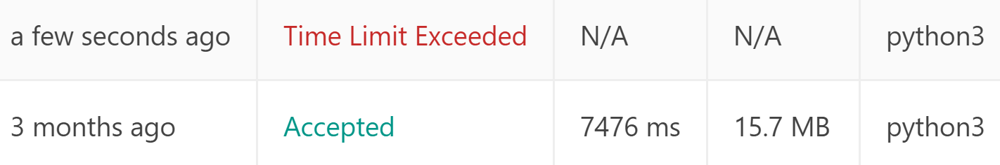Hard DifficultyLeetCode SolutionsPython

[LeetCode 839] Similar String Groups

Two strings X and Y are similar if we can swap two letters (in different positions) of X, so that it equals Y. Also two strings X and Y are similar if they are equal.

For example, "tars" and "rats" are similar (swapping at positions 0 and 2), and "rats" and "arts" are similar, but "star" is not similar to "tars""rats", or "arts".

Together, these form two connected groups by similarity: {"tars", "rats", "arts"} and {"star"}.  Notice that "tars" and "arts" are in the same group even though they are not similar.  Formally, each group is such that a word is in the group if and only if it is similar to at least one other word in the group.

We are given a list strs of strings where every string in strs is an anagram of every other string in strs. How many groups are there?

Example 1:

Input: strs = ["tars","rats","arts","star"]
Output: 2

Example 2:

Input: strs = ["omv","ovm"]
Output: 1

Constraints:

• 1 <= strs.length <= 100
• 1 <= strs[i].length <= 1000
• sum(strs[i].length) <= 2 * 104
• strs[i] consists of lowercase letters only.
• All words in strs have the same length and are anagrams of each other.

UNION FIND SOLUTION

Union find TLE solution
59 / 62 test cases passed.

class Solution:
def numSimilarGroups(self, A: List[str]) -> int:
self.uf = {}
def union(x, y):
self.uf[find(y)]=self.uf[find(x)]
def find(x):
self.uf.setdefault(x,x)
if x!=self.uf[x]:
self.uf[x] = find(self.uf[x])
return self.uf[x]
if len(set(A))==1:return 1
for a,b in itertools.combinations(A, 2):
dif =0
for aa,bb in zip(a,b):
if aa!=bb:
dif+=1
if dif>2:break
else:
union(a,b)
return len({find(a) for a in A})

Failed cases

“[“aaaaacedfb”,”aaaaacfbde”,”aaaaabecfd”,”aaaaaefdbc”,”aaaaafdecb”,”aaaaacefdb”,”…]'

We can do some trick to reduce the word size if they have the same character for a position.
If we do so, the above failed test case will become:

“[“cedfb”,”cfbde”,”becfd”,”efdbc”,”fdecb”,”cefdb”,”…]

as the first several letters for all words are the same.
We can do this trick naively, and still we will get TLE.
59 / 62 test cases passed.

class Solution:
def numSimilarGroups(self, A: List[str]) -> int:
self.uf = {}
def union(x, y):
self.uf[find(y)]=self.uf[find(x)]
def find(x):
self.uf.setdefault(x,x)
if x!=self.uf[x]:
self.uf[x] = find(self.uf[x])
return self.uf[x]
A_by_col = [new_a for new_a in zip(*A) if len(set(new_a))!=1]
if not A_by_col:return 1
for a,b in itertools.combinations(zip(*A_by_col), 2):
dif =0
for aa,bb in zip(a,b):
if aa!=bb:
dif+=1
if dif>2:break
else:
union(a,b)
return len({find(a) for a in zip(*A_by_col)})

Never give up, if we do this by sorting A firstly, and then only compare first A with last word A[-1] from the first position letter to last one. If they are the same, then all words are the same for this position. If not, we break. It is faster than the previous solution and got AC by lucky. I think if the same part is not putting at the beginning and it is randomly put, we will still get TLE.
AC solution (7484 ms)

class Solution:
def numSimilarGroups(self, A: List[str]) -> int:
self.uf = {}
def union(x, y):
self.uf[find(y)]=find(x)
def find(x):
self.uf.setdefault(x,x)
if x!=self.uf[x]:
self.uf[x] = find(self.uf[x])
return self.uf[x]
#***********trick to get it pass  ***************
A.sort()
valid_idx = []
for i in range(len(A)):
if A[-1][i]==A[i]:continue
else:
valid_idx += list(range(i, len(A)))
break
if not valid_idx:return 1
#*************************************************
for a,b in itertools.combinations(A, 2):
dif =0
for k in valid_idx:
if a[k]!=b[k]:
dif+=1
if dif>2:
break
else:
union(a,b)
return len({find(a) for a in A})

Updated April 6, 2020.
Thanks for @Mycloud to point out that this one still get TLE. Yes, I think this algorithm is not that fast and it got AC by lucky about 3 months ago. Please try to use some other faster algorithm and taking this one as reference.Follow-up question:

Why is UF so much faster than BFS, DFS for this problem?

0143
Previous Post

Next article